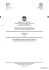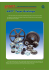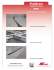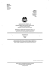# BAHAGIAN MATRIKULASI MATEMATIK

## Transcription

BAHAGIAN MATRIKULASI MATEMATIK
```QMo1611
QM()16/1
Mathematics
Paper 1
Semester
Matematik
Kertas 1
I
Semester I
2008t2009
2008/2009
2 hours
2 jam
&
-Y:
-r=-
BAHAGIAN MATRIKULASI
KEMENTERIAN PELAJARAN MALAYSIA
MATRIC ULATION DII/ISION
MINISTRY OF EDUCATION MALAYSIA
PEPERIKSAAN SEMESTER PROGMM MATRIKULASI
IATRICULATION P ROGRAMME EXAMINATION
MATEMATIK
Kertas 1
2 jam
JANGAN BUKA KERTAS SOALAN INISEHINGGA DIBERITAHU.
DO NOT OPEN IHIS BOOKLET UN'flLYOU ARE TOLD IO DO SO.
t
CHOW CHOON WOOI
Kertas soalan ini mengandungi 13 halaman bercetak.
This booklet consrsfs of 13 printed pages.
O Bahagian Matrikulasi
r
QM(}16/1
INSTRUCTIONS TO CANDIDATE:
This question booklet consists of 10 questions.
The
full marks allocated for each question or section is shown in the bracket
at the end
of
each question or section.
'
All
steps must be shown clearly.
Only non-programmable scientific calculator can be used.
Numerical answers can be given in the form of n, e, surd, fractions or correct to three
significant figures, where appropriate, unless stated otherwise in the question.
CHOW CHOON WOOI
3
QMo16/1
LIST OF MATHEMATICAL FORMULAE
Arithmetic Series:
T,=o+(n-t)a
s,=|lzr+(n_lal
Geometric Series:
T, = ar'-l
'.=+!
ror
r<l
Binomial Expansions:
(a +
(
I
"\ t-
b)' = o'
+(i)"".(;)"-u'
+-.
-.(:)"'
u' +. . + b',
where,e e N and
vlt
[,J-;G-4
(t+x)' =r+nx*"fu:') *, +...*n(n-l)":(n-r+l) x, +... for lxl<1
2!
rt
CHOW CHOON WOOI
5
QMo16/1
1.
(-2 r2*
Express
'
rQ
/!_;f-{
(t-x'fl+x)
in partial fractions.
[5 marks]
2.
The fifth term and the tenth term of a geometric series are 3125 and,243respectively.
(a)
Find the value of cornmon ratio, r of the series.
[3 marks]
(b)
Determine the smallest value
the sum of the
series.
first
r
of n
term and
such
S*
that
S-; S' <
0.02, where S, is',
s_
is the sum to
infinity of the geometric
[3 marks]
3.
Solve the equation 3log, 3 + 1og,
V;
=
+J
[7 marks]
4.
Determine the interval
of x satis\$ing the inequality lx+Zl>tO-x2
.
[7 marks]
,-
5.
The roots of the quadratic equation 2x2
(a)
Find the values
of az + p2
=4x-1
and. a3
are
p + aB3
a
and p.
.
[5 marks]
(b)
Form a new quadratic equation whose roots are (o
-Z)
ana (p -Z).
[5 marks]
CHOW CHOON WOOI
7
QMo16/1
,2
(a)
zl-zz
where
a
and
b
arereal numbers. Hence, determine
'
l-1-l
lzr-zzl
[5 marks]
(b)
Giventhat z=x + ry, where
complex conjugate
of z.
x
and
y aretherealnumbers and Z isthe
Find the positive values
of x and y
so that
12
-+
Zz -=5-t.
[6 marks]
1
(a)
6r).
The rth term of an arithmetic progression is (1+
sum of the
Find in terms of n, the
first n terms of the progression.
[4 marks]
(b)
(D
Showthat
I
=!(r-I)-'
"lg-x 3\ 9)
[3 marksl
I
(ii)
of (t- +)-' t,
I ei
ascending powers of x and state the range of values of x for which
Find the first three terms in the binomial expansion
this expansion is valid.
[3 marks]
(iii)
Find the first three terms in the expansion
'
powers
of
o, :( + x)
in ascending
"lg-x
x.
[3 marks]
CHOW CHOON WOOI
9
QMo16/1
I z 2 -:l
'
'1
(a) Given the matrice, , = [' , ,l and n=| , -r o ttnu Pe and
t, 2 2)
l.
Ll
L-3 0 3.1
8.
hence,
determine P-t .
r
[4 marks]
(b)
The following table shows the quantities (kg) and the amount paid (RM) for
the three types of items bought by three housewives in a supermarket.
Housewives
Sugar (kg)
Flour (kg)
Rice (kg)
Amount Paid (RM)
Aminah
3
6
J
a
16.s0
Malini
6
3
6
21.30
Swee Lan
J
6
6
2l.00
The prices in RM per kilogram (kg) of sugar, flour and rice are x,
y
and z
respectively.
(i)
F'orm a system of iinear equations from the above information and
write the system of linear equations in the form of matrix equation
AX=8.
[3 rnarks]
(ii)
Rewrite AX = B
P
above in the
form kPX: B, where
k is a constant.
k and hence find the values of x, y and z.
(
is the matrix in (a)
)
and,
A:
kP
Determine the value
of
[6 marks]
9.
Polynomial PG) = mxt -8xz +nx+6 can be divided exactly
the values
of m
and n. Using these values
of m
and
by *' -2x-3.
Find
n, factorize the polynomial
completely. Hence, solve the equation
3x4 -14x3
"
+llx2 + l6x-lz = a
using the polynomial P(.r).
[13 marks]
CHOW CHOON WOOI
11
QM016/1
10. Matrix I
is given
by
, = [: I ]'l
lz -3 -rl
(a)
Find
(i)
(it)
(iir)
the determinant
the minor
of
l,
of ,4 and
of A.
[9 marls]
(b)
Based on part (a) above,
f,ind
l-t . Hence, solve the simultaneous equations
Y+ z=12
5x+y
2x
-
z =9
-3y -1,
=i.
2
[6 marks]
END OF BOOKLET
CHOW CHOON WOOI
13
```

### Mathshammil### NSPT Taper Bushings